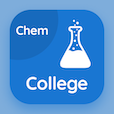Colleges Online Courses

College Chemistry Quizzes

College Chemistry Quiz PDF - Complete

# Charge to Mass Ratio of Electron Multiple Choice Questions p. 83

Study Charge to Mass Ratio of Electron multiple choice questions and answers, charge to mass ratio of electron quiz answers PDF 83 to study College Chemistry course online. Atomic Structure MCQ trivia questions, charge to mass ratio of electron Multiple Choice Questions (MCQ) for online college degrees. "Charge to Mass Ratio of Electron MCQ" PDF eBook: what is spectrum, ionization energies, kinetic molecular theory of gases, types of solids, charge to mass ratio of electron test prep for online bachelor degree programs.

"E/m value of electrons comes out to be" MCQ PDF: x1011, x1011, x1011, and x1011 for ACT test prep classes. Learn atomic structure questions and answers to improve problem solving skills for college entrance exams.

## Charge to Mass Ratio of Electron Questions and Answers MCQs

MCQ: E/m value of electrons comes out to be

x1011
x1011
x1011
x1011

MCQ: In crystalline solids, atoms and ions are arranged in

regular three dimensional pattern
irregular three dimensional pattern
simple two dimensions
one dimension

MCQ: Kinetic equation of gases was derived in

1876
1876
1857
1890

MCQ: The electron affinity of a hydrogen atom is

−73
−72
−71
−74

MCQ: The other source of rainbow other than the sun is

incandescent solids
soft solids
liquids
gases

### More Quizzes from College Chemistry Course